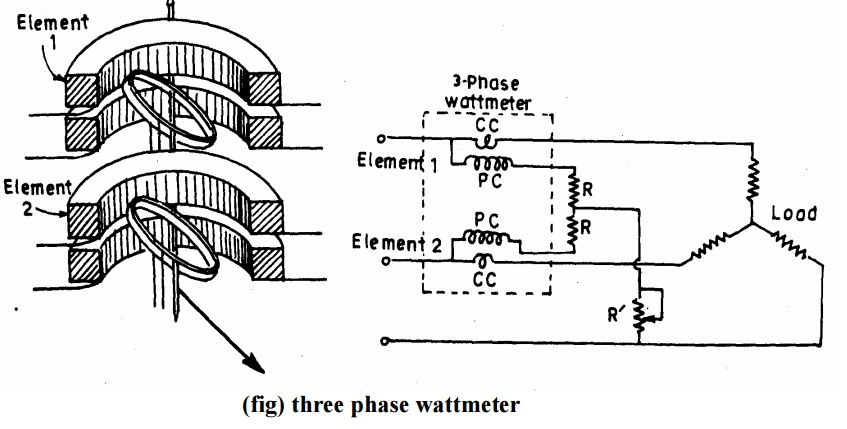Home | | Measurements and Instrumentation | Three Phase Wattmeters

# Three Phase WattmetersA dynamometer type three-phase wattmeter consists of two separate wattmeter movements mounted together in one case with the two moving coils mounted on the same spindle.

Three Phase Wattmeters

A dynamometer type three-phase wattmeter consists of two separate wattmeter movements mounted together in one case with the two moving coils mounted on the same spindle.

The arrangement is shown in Fig.

There are two current coils and two pressure coils.

A current  coil together with its pressure coil  is known as an element.

Therefore, a three phase wattmeter has two elements.

The connections of two elements of a 3 phase wattmeter are the same as that for two wattmeter method using two single phase wattmeter.

The torque on each element is proportional to the power being measured by it. The total torque deflecting the moving system is the sum of the deflecting torque of’ the two elements.

Hence the total deflecting torque on the moving system is proportional to the total Power.

In order that a 3 phase wattmeter read correctly, there should not be any mutual interference between the two elements.

A laminated iron shield may be placed between the two elements to eliminate the mutual effects.Study Material, Lecturing Notes, Assignment, Reference, Wiki description explanation, brief detail

Related Topics